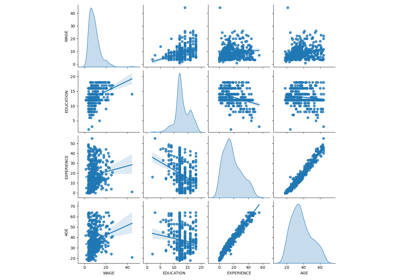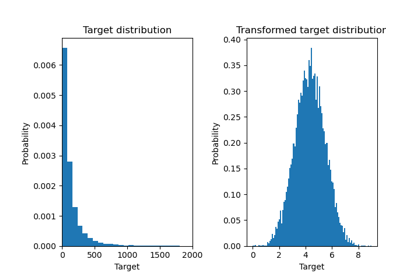# sklearn.metrics.median_absolute_error¶

sklearn.metrics.median_absolute_error(y_true, y_pred, *, multioutput='uniform_average', sample_weight=None)[source]

Median absolute error regression loss.

Median absolute error output is non-negative floating point. The best value is 0.0. Read more in the User Guide.

Parameters:
y_truearray-like of shape (n_samples,) or (n_samples, n_outputs)

Ground truth (correct) target values.

y_predarray-like of shape (n_samples,) or (n_samples, n_outputs)

Estimated target values.

multioutput{‘raw_values’, ‘uniform_average’} or array-like of shape (n_outputs,), default=’uniform_average’

Defines aggregating of multiple output values. Array-like value defines weights used to average errors.

‘raw_values’ :

Returns a full set of errors in case of multioutput input.

‘uniform_average’ :

Errors of all outputs are averaged with uniform weight.

sample_weightarray-like of shape (n_samples,), default=None

Sample weights.

New in version 0.24.

Returns:
lossfloat or ndarray of floats

If multioutput is ‘raw_values’, then mean absolute error is returned for each output separately. If multioutput is ‘uniform_average’ or an ndarray of weights, then the weighted average of all output errors is returned.

Examples

>>> from sklearn.metrics import median_absolute_error
>>> y_true = [3, -0.5, 2, 7]
>>> y_pred = [2.5, 0.0, 2, 8]
>>> median_absolute_error(y_true, y_pred)
0.5
>>> y_true = [[0.5, 1], [-1, 1], [7, -6]]
>>> y_pred = [[0, 2], [-1, 2], [8, -5]]
>>> median_absolute_error(y_true, y_pred)
0.75
>>> median_absolute_error(y_true, y_pred, multioutput='raw_values')
array([0.5, 1. ])
>>> median_absolute_error(y_true, y_pred, multioutput=[0.3, 0.7])
0.85


## Examples using sklearn.metrics.median_absolute_error¶Common pitfalls in the interpretation of coefficients of linear models

Common pitfalls in the interpretation of coefficients of linear modelsEffect of transforming the targets in regression model

Effect of transforming the targets in regression model